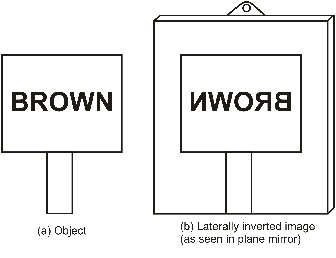# 10th Physics | Light | Plane Mirror

## Plane Mirror

Any smooth, highly polished reflecting surface is called a mirror. A plane mirror is a highly polished plane surface.

### CHARACTERISTICS OF IMAGE FORMED BY PLANE MIRRORS

Following are the important characteristics of image formed by plane mirrors.Image formation by a plane mirror

Figure 1

1.    The image formed in a plane mirror is always virtual. Such an image cannot be taken on a screen. (figure 1)

2.    The image formed in a plane mirror is always erect. It is the same side up as the object. (figure 1)

3.    The image in a plane mirror is of the same size as the object. (figure 1)

4.    The image formed by a plane mirror is at the same distance behind the mirror as the object is in front of the mirror i.e. image distance is always equal to the object distance in a plane mirror. (figure 1)

5.    The image formed in a plane mirror is laterally inverted i.e. the left side of the object becomes the right side of the image and vice-versa as shown in the figure 2.Figure 2: Diagram to show lateral inversion

### Question:     If an object is placed at a distance of 10 cm in front of a plane mirror, how far would it be from its image?

Solution:

The object would be at a distance of 10 cm + 10 cm = 20 cm from its image.

### Question:     State four characteristics of the image formed in a plane mirror.

Solution:

The image formed in a plane mirror is :

(a) always virtual and erect.

(b) of the same size as the object.

(c) at the same distance behind the mirror as the object is in front of it.

(d) laterally inverted (sideways reversed).

This site uses Akismet to reduce spam. Learn how your comment data is processed.